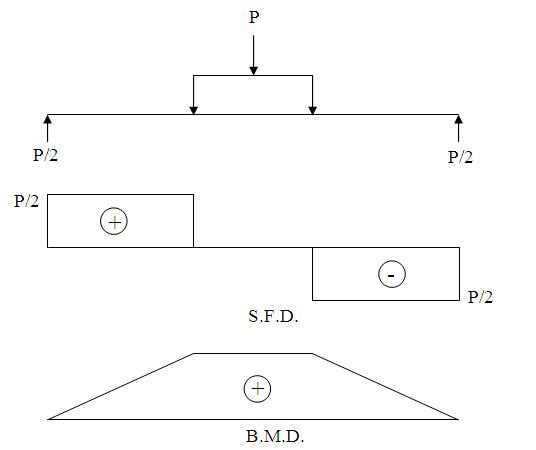Foundation, Concrete and Earthquake Engineering

### STUDY ON STRENGTH OF CONCRETE USING VARIOUS COARSE AGGREGATES, PART-18

5.4.3.4 Calculation

If the fracture initiates in the tension surface within the middle third of the span length, we can calculate the modulus of rupture as follows:

R=Pl/bd2

where

R= modulus of rupture, psi
P= maximum applied load indicated by the testing machine, lbf
l = span length, inch
b= average width of specimen, inch
d= average depth of specimen, inch

5.4.4 Diagrams for shear strengthFig No. 5.3: Shear force and Bending moment diagram.